# How to Calculate and Solve for Phase Lag | Electromagnetic Method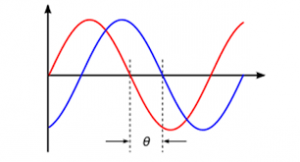The image above represents phase lag.

To compute for phase lag, three essential parameters are needed and these parameters are Frequency (f), Inductance (L) and Resistance (R).

The formula for calculating the phase lag:

φ = tan-1 (2πfL/R)

Where:

φ = Phase Lag
f = Frequency
L = Inductance
R = Resistance

Let’s solve an example;
Find the phase lag when the frequency is 20, the inductance is 32 and the resistance is 18.

This implies that;

f = Frequency = 20
L = Inductance = 32
R = Resistance = 18

φ = tan-1 (2πfL/R)
φ = tan-1 (2π(20)(32) / 18)
φ = tan-1 (4021.23 / 18)
φ = tan-1 (223.40)
φ = 89.74°

Therefore, the phase lag is 89.74°.

Nickzom Calculator – The Calculator Encyclopedia is capable of calculating the phase lag.

To get the answer and workings of the phase lag using the Nickzom Calculator – The Calculator Encyclopedia. First, you need to obtain the app.

You can get this app via any of these means:

To get access to the professional version via web, you need to register and subscribe for NGN 1,500 per annum to have utter access to all functionalities.
You can also try the demo version via https://www.nickzom.org/calculator

Apple (Paid) – https://itunes.apple.com/us/app/nickzom-calculator/id1331162702?mt=8
Once, you have obtained the calculator encyclopedia app, proceed to the Calculator Map, then click on Geology under Add-on.Now, Click on Electromagnetic Method under GeologyNow, Click on Phase Lag under Electromagnetic Method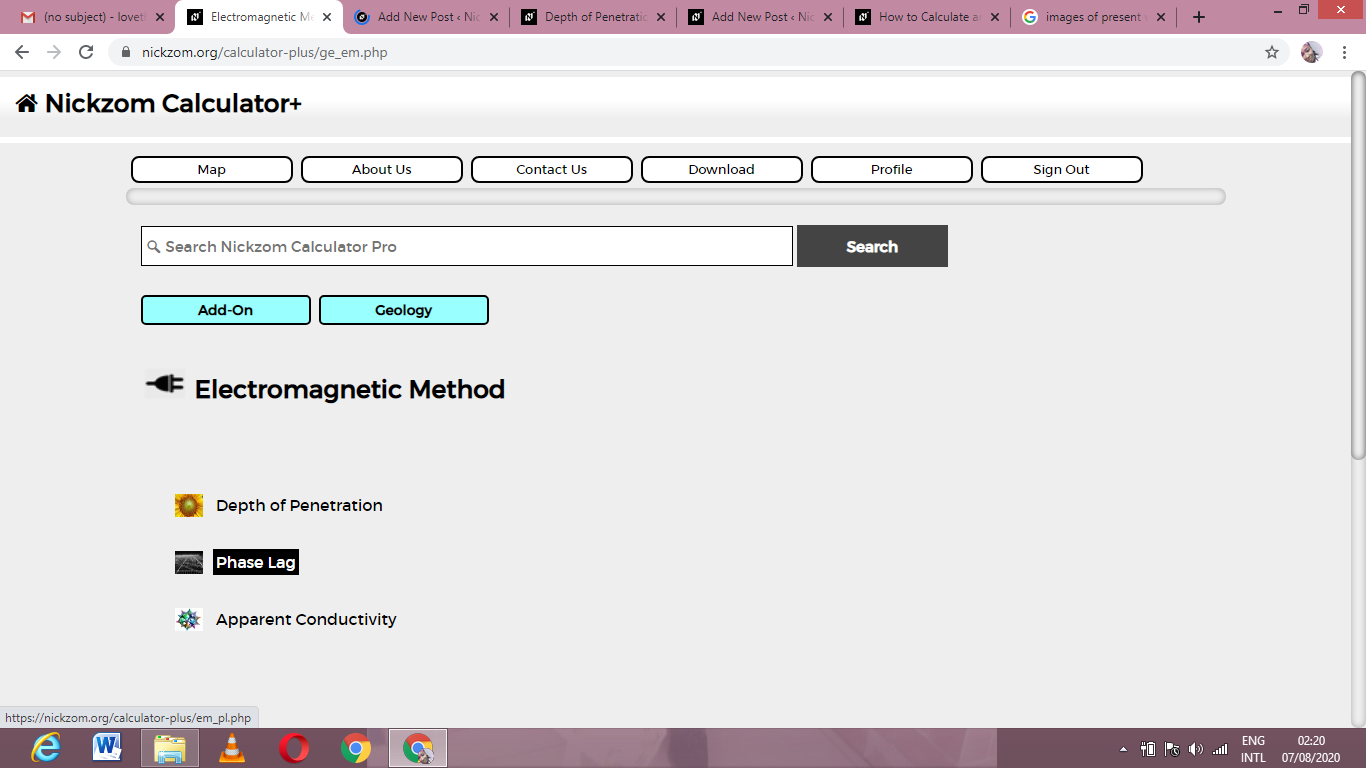The screenshot below displays the page or activity to enter your values, to get the answer for the phase lag according to the respective parameters which are the Frequency (f), Inductance (L) and Resistance (R).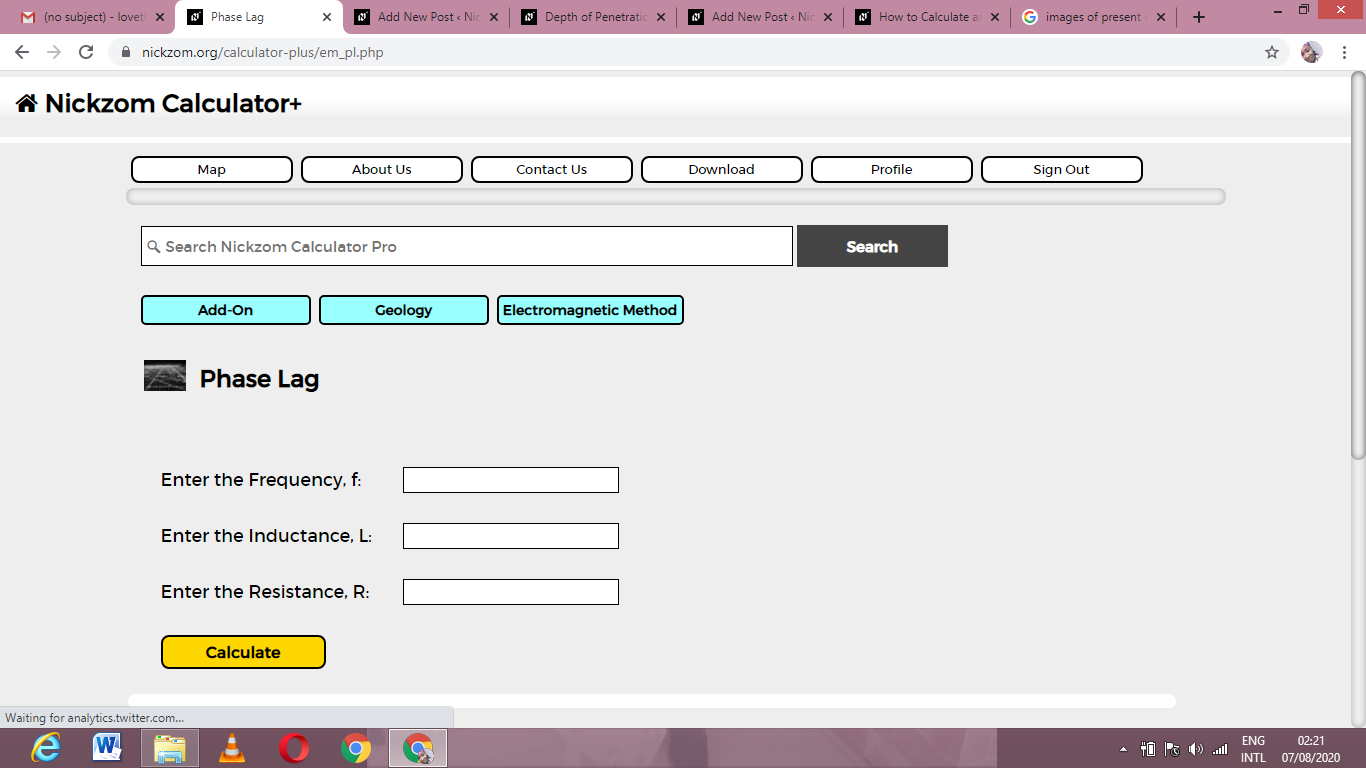Now, enter the values appropriately and accordingly for the parameters as required by the Frequency (f) is 20, Inductance (L) is 30 and Resistance (R) is 18.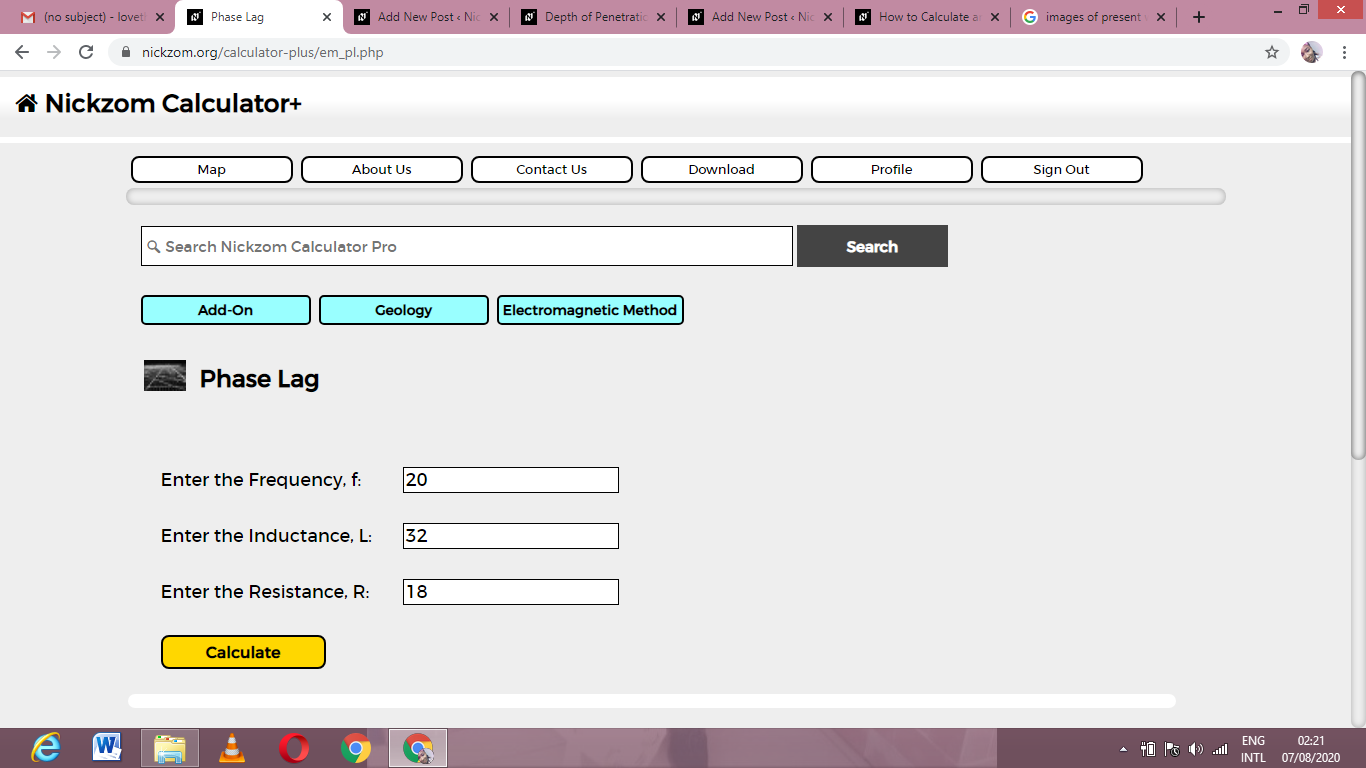Finally, Click on Calculate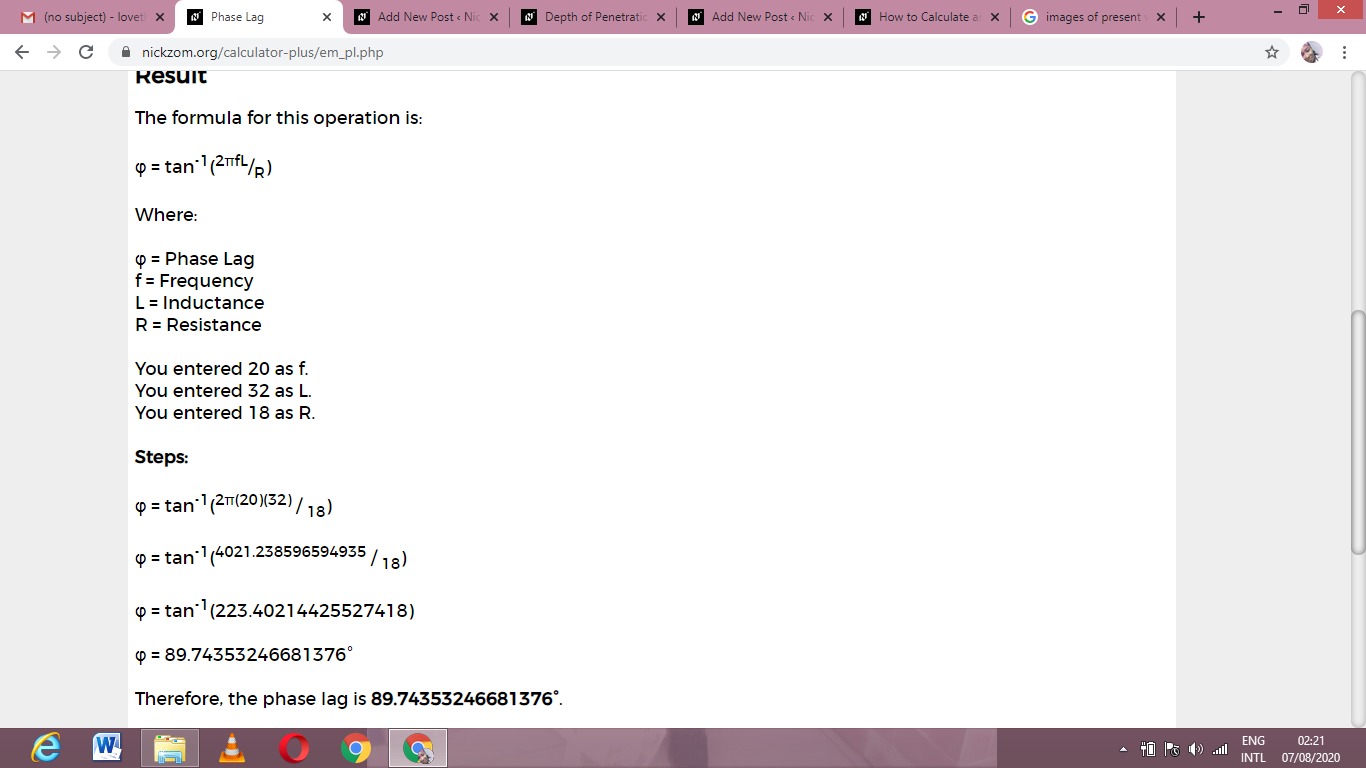As you can see from the screenshot above, Nickzom Calculator– The Calculator Encyclopedia solves for the phase lag and presents the formula, workings and steps too.